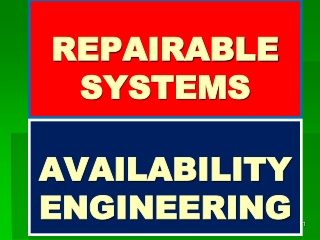DownloadDownload PresentationREPAIRABLE SYSTEMS

# REPAIRABLE SYSTEMS

Télécharger la présentation## REPAIRABLE SYSTEMS

- - - - - - - - - - - - - - - - - - - - - - - - - - - E N D - - - - - - - - - - - - - - - - - - - - - - - - - - -
##### Presentation Transcript

1. REPAIRABLESYSTEMS AVAILABILITYENGINEERING

2. A Single Repairable Component With Failure Rate λand Repair Rate μ The Concept of AVAILABILITY The component may exist in one of Two States SO (Working) and S1 (Failing).It transits from SO to S1 by Failure and back from S1 to SO by Repair SO λ μ S1 STATE GRAPH TRANSITION MATRIX It is required to evaluate Probabilities PO and P1 Of the component being in states SO and S1 Prof. Ahmed Farouk Abdul Moneim

3. TRANSITION EQUATIONS Multiply both sides by

4. Then At Steady state as t tends to infinity, the component(system) will be AVAILABLE with probability Prof. Ahmed Farouk Abdul Moneim

5. Another Approach to AVAILABILITY As t tends to infinity, Po = Availability = const and dPo / d t =0, then λ=1/MTBF μ=1/MTTR Therefore

6. Availability of A System with One Unit Standby Prof. Ahmed Farouk Abdul Moneim

7. This system could exist in one of three states So , S1 and S2: So state of system operating with probability Po S1 state of system with the main component failed and switched to the standby unit to operate ( probability of being in this state P1, S2 State of having both units main and standby failed with probability P2 λ λ S1 S2 SO STATE GRAPH 2 μ Two Repair gangs are available μ Continuity Equation Apply HARMONIC BALANCE to states So and S2 Substitute in (1), we find

8. In a metal cutting workshop, there are two different machines: Machine A: a mechanical cutting with capacity of 10 tons per hour. The machine has a constant failure rate of 3 failures per month and mean repair time of 5 days Machine B: a gas cutting machine with capacity of 20 tons per hour . The machine has a constant failure rate of 4 failures per month and mean repair time of 3 days. Evaluate the EFFECTIVE CAPACITY of the workshop. _____________________________________________________________________ The Workshop could exist in one of the following states So Both machines are working. S1 Mechanical Cutting failed and gas Cutting works S2 Gas Cutting failed and Mechanical Cutting works S3 Both failed SO λ g λ m μ g μ m S1 S2 μ g μ m S3 Required to find the FOUR probabilities, Po, P1, P2, P3 The first equation: Po + P1 + P2 + P3 = 1 ………………………….(1) The other THREE equation will be obtained by applying HARMONIC BALANCE to the three states So, S1, S2 as follows: λ g λ m Prof. Ahmed Farouk Abdul Moneim

9. Apply to So Apply to S1 Apply to S2 Omitting P3 from the two equations (3) and (4), we get Consider (2) and (5) to omit P2, we find Substitute in (2) Substitute in (3)

10. Substitute in (1), we get Po + P1 + P2 + P3 =1……….(1) Effective Capacity = (20+10)*Po + 20*P1 + 10 * P2 Prof. Ahmed Farouk Abdul Moneim

11. Evaluation of the RELIABILITY of the above considered REPAIRABLE SYSTEM Consider the following three equations in the three unknowns: Apply Laplace Transform on the above three equations Prof. Ahmed Farouk Abdul Moneim

12. Where, Is the Laplace Transform of Pm. Therefore, Solving the above system of algebraic equations Prof. Ahmed Farouk Abdul Moneim

13. At t = 0, PO =1 : Therefore, A is the AVAILABILITY λ=1/MTTF μ=1/MTTR Therefore Prof. Ahmed Farouk Abdul Moneim